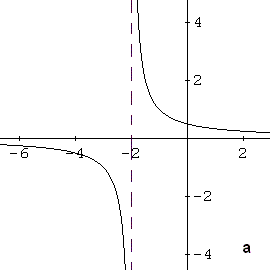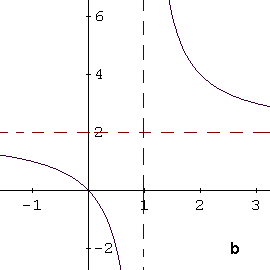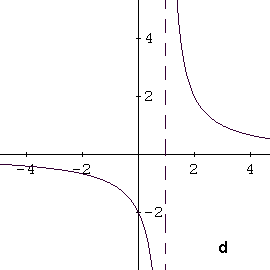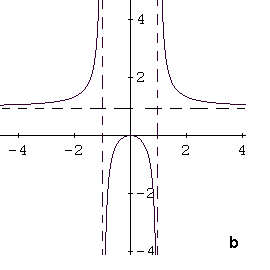# Graphs of Rational Functions - Tutorials

This is a tutorial on identifying the graph of a rational function given by its formula.

Four graphs are given and only one of them is the possible graph corresponding to the given function. The vertical and horizontal asymptotes are shown in broken lines.  Examples with detailed solutions are presented below.

You may want to go through tutorials on graphing and rational functions before starting this tutorial.

Example 1 : Identify the graph of the function f(x) = 2x/(x - 1)Solution to Example 1:

The given function f(x) = 2x/(x - 1) has a vertical asymptote at x = 1 and a horizontal asymptote at y = 2. If you examine the 4 graphs given above only graph b has a vertical asymptote at x = 1 and a horizontal asymptote at y = 2.

Example 2 : Identify the graph of the function f(x) = x2 / (x2 - 1)Solution to Example 2:

The given function f(x) = x2 / (x2 - 1) has 2 vertical asymptotes at x = 1 and x = -1 and a horizontal asymptote at y = 1. If you examine the 4 graphs given above only graph b has these characteristics.